Area of circle

Chapter 9 Class 7 Perimeter and Area
Concept wise

For a circle with radius r

Area of circle will be

Area of circle = 𝜋 r 2

Here,

𝜋  can be taken as

𝜋 = 3.14 or 𝜋 = 22/7

Let’s take some examples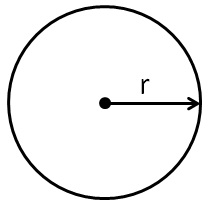## Find area of circle of radius 2 cm by counting squares , and also by formula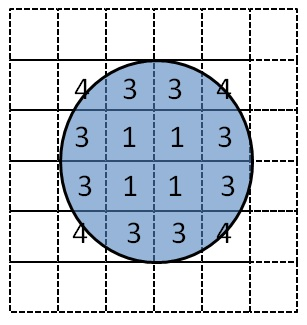Covered Area Number Area 1 Fully - filled squares 4 1 × 4 = 4 2 Half - filled squares 0 1/2 × 0 = 0 3 More than Half - filled squares 8 1 × 8 = 8 4 Less than Half - filled squares 4 0 × 4 = 0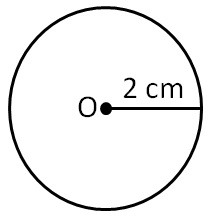Area = 4 + 0 + 8 + 0

= 12 cm 2

By formula of area of circle

Area = 𝜋r 2

= 3.14 × (2) 2

= 3.14 × 4

= 314/100 × 4

= 1256/100

= 12.56 cm 2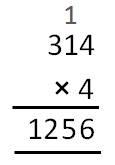So, finding area by formula is more accurate

#### Find area of a circle with radius of 3.5 cm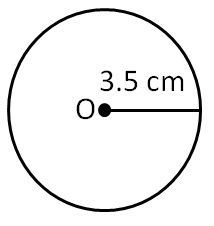Radius = r = 3.5 m

Area = 𝜋r 2

= 𝜋 (3.5) 2

= 22/7 × (35/10) 2

= 22/7 × (7/2) 2

= 22/7 × 7/2 × 7/2

= 11/7 × 7/1 × 7/2

= 11/1 × 1 × 7/2

= 77/2

= 38.5 cm 2

∴ Area is 38.5 cm 2

#### Find area of a circle whose radius of 7 cm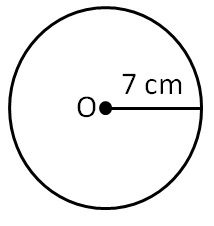Radius = r = 7 cm

Area = 𝜋 r 2

= 22/7 × (7) 2

= 22/7 × 7 × 7

= 22 × 7

= 154 cm 2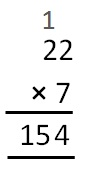∴ Area is 154 cm 2

Find area of a circle with diameter 21 cm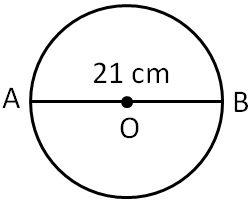Diameter = 21 cm

= 21/2 cm

Now,

Area = 𝜋 r 2

= 22/7 × (21/2) 2

= 22/7 × 21/2 × 21/2

= 11/7 × 21/1 × 21/2

= 11/1 × 3 × 21/2

= (33 × 21)/2

= 693/2

= 346.5 cm 2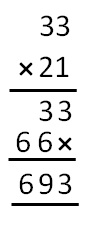∴ Area is 346.5 cm 2

#### Find area of a circle with diameter 42 cm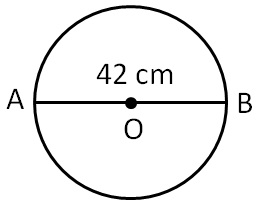Diameter = 42 cm

= 42/2 cm

= 21 cm

Now,

Area = 𝜋 r 2

= 22/7 × (21) 2

= 22/7 × 21 × 21

= 22/1 × 3 × 21

= 66 × 21

= 1386 cm 2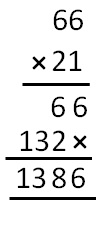∴ Area is 1386 cm 2

#### Find area of a circle with radius 5 cm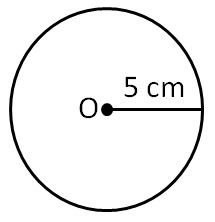= 5 cm

Now,

Area = 𝜋 r 2

= 3.14 × (5) 2

= 3.14 × 25

= 314/100 × 25

= 314/20 × 5

= 314/4 × 1

= 157/2

= 78.5 cm 2

∴ Area is 78.5 cm 2

#### Find the area of a circle with diameter 30 cm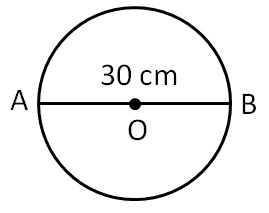Diameter = 30 cm

= 30/2 cm

= 15 cm

Now,

Area = 𝜋 r 2

= 3.14 × (15) 2

= 3.14 × 225

= 314/100 × 225

= 314/20 × 45

= 157/10 × 45

= 7065/10

= 706.5 cm 2

∴ Area = 706.5 cm 2

Learn in your speed, with individual attention - Teachoo Maths 1-on-1 Class